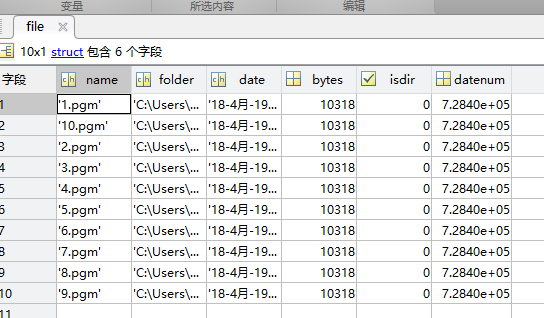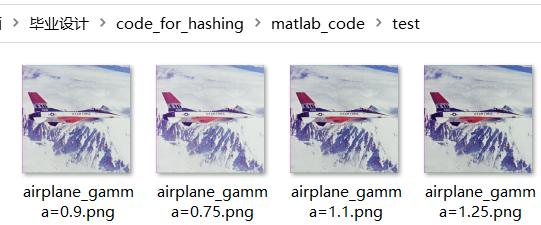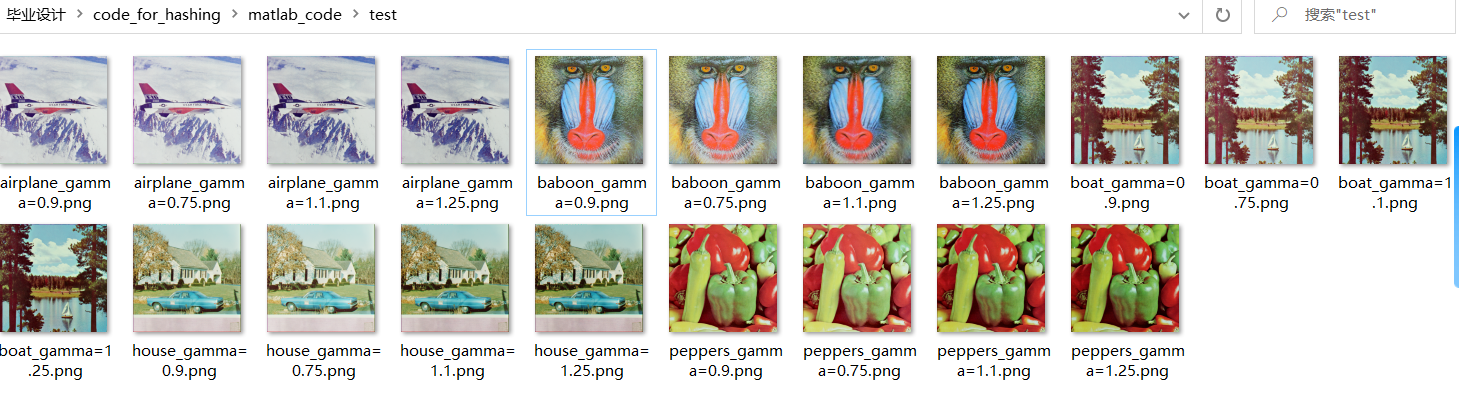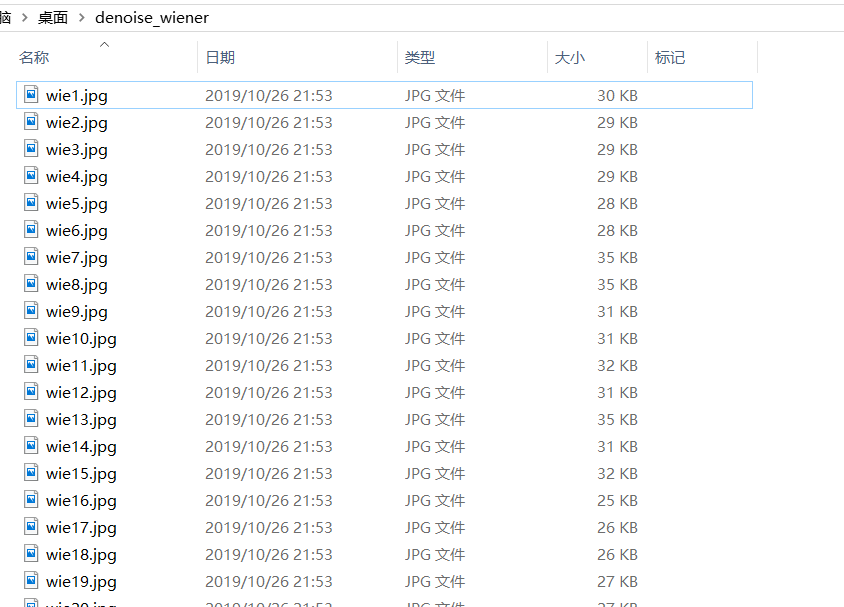•批量读取图片
• matlab批量读取图片

千次阅读 2019-10-06 20:12:27

• 如果文件夹中的图片已经编号

预置知识：

1. cell元胞数组。cell是一种特殊的数据结构，这个数组中的数据类型是任意的
>> C={1,'cell';{1,2,3},{}}

C =

2×2 cell 数组

{[     1]}    {'cell'  }
{1×3 cell}    {0×0 cell}

>> cell(2,3)

ans =

2×3 cell 数组

{0×0 double}    {0×0 double}    {0×0 double}
{0×0 double}    {0×0 double}    {0×0 double}

使用cell命令可以声明cell数组，声明格式与zeros()函数，ones（）函数完全相同。

注意cell数组是用大括号括起来的，可以直接声明，也可以使用C{index}的格式进行索引

2.strcat（）函数

用来串接字符数组。可以串接一般字符数组，也可以串接元胞数组。

如果两个字符数组都只有一个字符串，那么好说

>> s1='lwz';
>> s2='hhh';
>> strcat(s1,s2)

ans =

'lwzhhh'

如果是每个字符数组有多个元素呢？

>> str1={'lwz','hhh'};
>> str2={'lbw','nb'};
>> strcat(str1,str2)

ans =

1×2 cell 数组

{'lwzlbw'}    {'hhhnb'}

可以看到是“对位串接”

现在开始读取图片：

n=10;

I=cell(1,n);
for i=1:n
imName=strcat(num2str(i),'.pgm');
end
• 如果图片没有编号

cd('C:\Users\22926\Desktop\图像工程基础\s1');
file=dir('*.pgm');
[k,len]=size(file);
I=cell(k,1);
for i=1:k
name=file(i).name;
figure(i);
imshow(I{i});
end

注：

• cd命令的含义是切换当前路径，与DOS命令一样
• dir命令（）：
• Matlab使用dir函数获得指定文件夹下的所有子文件夹和文件,并存放在在一种为文件结构体数组中.（struct，规模是k*6，包含六个属性）• dir函数可以有调用方式为：

dir('.')列出当前目录下所有子文件夹和文件
dir('G:\Matlab')列出指定目录下所有子文件夹和文件
dir('*.m')列出当前目录下符合正则表达式的文件夹和文件
• 每次imshow()之前加一个figure(i)，可以搞出多个图片显示窗口
展开全文• ·读取一个文件夹内的所有图片 ·依次修改每张图的尺寸（也可以进行其他的图像处理） ·批量保存图像 代码为： file_path = '.\RGB25\';% 图像文件夹路径 img_path_list = dir(strcat(file_path,'25_*.tif')); save...

·批量保存图像

代码为：

file_path =  '.\img_file\';% 图像文件夹路径
img_path_list = dir(strcat(file_path,'img_*.tif'));
save_path = '.\new_img0729\'  %另存为新的文件夹中

%dir 列出当前文件夹中的文件信息
img_num = length(img_path_list)%获取图像总数量
fprintf('正在读取的图像为：\n');
if img_num > 0 %有满足条件的图像
for j = 1:img_num %逐一读取图像
img_name = [file_path,'img_',int2str(j),'.tif'];
fprintf('第%02d个：%s\n',j,img_name);
B = imresize(pitch,[600 600]);
imwrite(B,strcat(file_path,'img_',int2str(j),'.tif'),'resolution',[600,600])
%如果选用file_path则覆盖原文件夹
%选用save_path则保存在新文件夹中
end
end

展开全文matlab 图像批处理 批量读取 批量保存
• xml中包含的是图片中缺陷区域的坐标，用matlab读取读取其中的信息，并批量读取图片，将缺陷区域保留，其余置黑，最后将处理后的图片保存到其他文件夹中xml文件读取
• end end 参考 MATLAB批量读取一个文件夹下的图片 how to load and read image sequences in order in matlab Gamma correction 伽马校准及 matlab 实现 matlab imwrite写入指定文件夹

处理1张图片，获取4幅不同伽马校正图像

实验结果实验代码

get_4_pictures_gamma_correction.m

%----批量处理伽马校正---
clc;clear;
name = 'airplane.png';
prefix = name(1:end-4); % 去掉name后面的.png
% figure,imshow(I);
gammaCorrection(name,1,0.75); %自定义函数

gamma_list = [0.75, 0.9, 1.1, 1.25];
for i = 1:length(gamma_list)
gamma = gamma_list(i);
s = gammaCorrection(name,1,gamma);
str0='D:\user\user\毕业设计\code_for_hashing\matlab_code\test\';
str1= [prefix,'_','gamma=',num2str(gamma)];%字符串拼接
str2 = '.png';
save_path=[str0,str1,str2]; % 字符串拼接
imwrite(s,save_path);
end

gammaCorrection.m

function [s]=gammaCorrection(name, a, gamma)
r=im2double(r);
s = a * (r .^ gamma);
%  imwrite(s,'airplane_gamma=0.75.jpg','jpg');
subplot (1 ,2 ,1), imshow(r), title('Original');
subplot (1 ,2 ,2), imshow(s), title(sprintf('Gamma: %0.2f',gamma));
end

批量读取图片模板

来源于参考1

file_path = 'D:\user\user\毕业设计\code_for_hashing\pictures_5_csv\';% 图像文件夹路径
img_path_list = dir(strcat(file_path,'*.png'));%获取该文件夹中所有png格式的图像
img_num = length(img_path_list)%获取图像总数量
I=cell(1,img_num);
if img_num > 0 %有满足条件的图像
for j = 1:img_num %逐一读取图像
image_name = img_path_list(j).name;% 图像名
I{j}=image;
fprintf('%d %d %s\n',i,j,strcat(file_path,image_name));% 显示正在处理的图像名
%图像处理过程 省略
%这里直接可以访问细胞元数据的方式访问数据

end
end

批量处理多张图片，分别获取4幅伽马校正图像

实验结果

img_num =

5

4 1 D:\user\user\毕业设计\code_for_hashing\pictures_5_csv\airplane.png
4 2 D:\user\user\毕业设计\code_for_hashing\pictures_5_csv\baboon.png
4 3 D:\user\user\毕业设计\code_for_hashing\pictures_5_csv\boat.png
4 4 D:\user\user\毕业设计\code_for_hashing\pictures_5_csv\house.png
4 5 D:\user\user\毕业设计\code_for_hashing\pictures_5_csv\peppers.png实验代码

file_path = 'D:\user\user\毕业设计\code_for_hashing\pictures_5_csv\';% 图像文件夹路径
img_path_list = dir(strcat(file_path,'*.png'));%获取该文件夹中所有png格式的图像
img_num = length(img_path_list)%获取图像总数量
I=cell(1,img_num);
if img_num > 0 %有满足条件的图像
for j = 1:img_num %逐一读取图像
image_name = img_path_list(j).name;% 图像名
I{j}=image;
fprintf('%d %d %s\n',i,j,strcat(file_path,image_name));% 显示正在处理的图像名
%图像处理过程
get_image_sequences_of_gamma_correction(file_path,image_name);
%这里直接可以访问细胞元数据的方式访问数据

end
end

get_image_sequences_of_gamma_correction.m

%----批量处理伽马校正---
function get_image_sequences_of_gamma_correction(path,name)

prefix = name(1:end-4); % 去掉name后面的.png
% figure,imshow(I);
gamma_list = [0.75, 0.9, 1.1, 1.25];
for i = 1:length(gamma_list)
gamma = gamma_list(i);
file_name = [path,name];
s = gammaCorrection(file_name,1,gamma);
str0='D:\user\user\毕业设计\code_for_hashing\matlab_code\test\';
str1= [prefix,'_','gamma=',num2str(gamma)];%字符串拼接
str2 = '.png';
save_path=[str0,str1,str2]; % 字符串拼接
imwrite(s,save_path);
end

end

参考

展开全文• Matlab批量读取，处理及保存图片

千次阅读 多人点赞 2019-10-28 14:22:34
最近想用MATALB对图片进行批量处理，之前都是用python比较多点，然后发现Matlab批量处理图片相对于python还是有点麻烦的，网上搜了下教程，也感觉有些麻烦，后来自己摸索了下，感觉有种方法还是不错的，现在与大家...

最近想用MATALB对图片进行批量处理，之前都是用python比较多点，然后发现Matlab批量处理图片相对于python还是有点麻烦的，网上搜了下教程，也感觉有些麻烦，后来自己摸索了下，感觉有种方法还是不错的，现在与大家分享下。

Input_path = 'C:\Users\ASUS\Desktop\LDCT_NOISE\';
Output_path='C:\Users\ASUS\Desktop\denoise_wiener\';
namelist = dir(strcat(path,'*.jpg'));  %获得文件夹下所有的 .jpg图片
len = length(namelist);
for i = 1:len
name=namelist(i).name;  %namelist(i).name; %这里获得的只是该路径下的文件名
K2=wiener2(I,[5 5]); %对图片进行去噪处理
imwrite(K2,[Output_path,'wie',int2str(i),'.jpg']); %完整的图片存储的路径名  并将整形的数字
转换成字符串
end

最后处理后保存图片的形式如下这里需要注意的是：

1.路径结尾要加上 "/"

2.namelist(i).name 只能获取文件的名字，就像 1.jpg ，并不能获得完整的路径名，所以读取图片的时候要特别注意将之前的路径添加一起

3.保存的时候，可以使用[ ],将图片保存到自己想要存储的位置，以及图片的名字，并且需要将循环变量 i 转换成字符串。

展开全文批量读取图片 Matlab 图像处理 wiener去噪
• matlab批量读取图片代码PyTorch-ARCNN 这是为ARCNN编写的非官方测试专用脚本。 它加载预训练的权重（.m）并在PyTorch中执行。 绝对没有培训功能。 有关详细信息，请访问论文作者的网站：。 这可以作为其他图像处理...
• 从一个文件夹下批量读取指定类型或者所有类型的文件，如所有的jpg图片，文件夹等，根据个人设置来获取读取结果，自己加限定，目前里面的实例是读取jpg图片matlab
• matlab批量读取图片代码mxnet查找小脸 这是的MXNet的仅推论实现。 有关更多信息，请参见以下参考： "Finding Tiny Faces." Peiyun Hu, Deva Ramanan arXiv preprint arXiv:1612.04402, 2016. 入门 下载原作者的模型 ...
• matlab批量读取文件夹中图片

千次阅读 2020-06-25 14:56:51
matlab批量读取文件夹中图片 file_path ='E:\graduate_student\Information_Hiding_CS_code\pictureDB\misc\';% 图像文件夹路径（注意misc文件夹后有一个斜杠） img_path_list = dir(strcat(file_path,'*.Tiff'));%...matlab
• matlab如何生成数据dat文件_数学_自然科学_专业资料。第一种方法:sav...matlab中读取txt中数据 2页 1... 1页 2下载券 matlab批量读写数据 2页 1下载券 ......用MATLAB生成DAT文件 f1=10;f2=100;fs=400;N=400...supp...
• matlab批量读取照片

千次阅读 2018-11-08 11:27:06
相信这个问题应该是每一个刚刚接触图像处理的小伙伴们在一开始最需要掌握的技能，否则一张一张的读取图片费时费力，并且遇到大型的项目工程，成百上千张图片一张一张进行读取也是非常不可行的。所以这里也为我自己...
• 分享一个能自动读取路径下指定类型图像的自写函数。...每次需要批量读取图片时，都要根据文件命名设计for循环实现，有时图片命名还没有规则就很难受。所以分享一种能自动读取路径下指定类型图像的函数。matlab DICOM图像
• 做图像处理实验，一般要对多张数据集进行读取、处理、以及数据和图像的保存（figure图像和去噪图像保存的几种方法） 本文列出具体代码的功能如下：1、从文件夹中读取图像数据（图像统一按顺序命名I1.bmp）2、对图像...matlab
• Matlab批量读取文件夹内图片

万次阅读 多人点赞 2018-07-06 10:30:20
Matlab读取文件夹里所有的文件image = dir('C:\images\*.*');files = dir(fullfile('C:\\Users\\Administrator\\Desktop\\sd\\','*.jpg')); lengthFiles = length(files); for i = 1:lengthFiles; Img = imread...
• matlab图片批量读取

万次阅读 多人点赞 2014-09-28 20:37:11
指定路径下 单个文件夹data中所有图像 file_path = '.\data\';% 图像文件夹路径 img_path_list = dir(strcat(file_path,'*.jpg'));%获取该文件夹中所有jpg格式的图像 img_num = length(img_path_list);%获取图像总...
• 图像处理过程中，经常会对图片的大小进行修改，传统的人工修改的速度较慢，这里利用MATLAB实现对多幅图像大小的修改，并且可以自定义所要修改图片的输出大小
• matlab批量读取处理文件

千次阅读 2021-04-14 21:23:49
matlab需要读取一个文件夹下的多个子文件夹中的某种指定格式的文件，该如何批量操作呢？ 首先，先介绍几个matlab中常用的函数 1. dir() 2. length() 3. isequal() 4. fullfile() 参考： ...matlab
• savepath='F:\matlabwork\Target image\blank1'; for i=0:10 x=b+2.5*d+i*7*d; rect=[a+(7*i-4)*d,m-1.5*d,3*d,3*d]; ... A=imcrop(im,rect);... imwrite(A,[savepath,num2str(i'%02d'),'.bmp...%将处理后的图片保存...
• % 如果你的图片命名方式是1.bmp 2.bmp....... clear;clc; file_path = '你的需要处理的图片的文件夹路径\'; img_path_list = dir(strcat(file_path,'*.bmp')); img_num = length(img_path_list); if img_num > 0 ...
• matlab批量读取并显示图片

千次阅读 2012-08-07 15:09:30
n=127; I=cell(1,n); for i=1:n  imageName=strcat('D:\img\',num2str(i),'.jpg');  I{i} = imread(imageName);   figure(1);  imshow(I{i});  endmatlab
• 最近在做图像处理的相关项目中涉及到文件的批量处理，个人觉得批量保存和读取文件简单而且实用，现以图片为例将其方法分享一下。 一、思想：在批量保存和读取文件时，为了方便固然是将文件名按某种规律进行保存和...机器学习 matlab
• MATLAB批量读取路劲下图像》的附带代码。内容文中可以，没积分可直接到https://blog.csdn.net/xsz591541060/article/details/107280266中复制即可。
• 看完标题，我们可以把这个任务当成两个子任务来实现：一是先批量读取指定文件夹下的图像，二是对读取的每一 帧图形裁剪；再就是如何设置，才可以裁剪出多张指定的大小？三是如何对处理后的图像进行批量保存?现在让...matlab 深度学习数据增强
• matlab批量读取同一目录下的txt文件

万次阅读 多人点赞 2018-12-05 20:19:09
1.MATLAB中一次导入一个txt文件或者其它数据文件很简单，直接通过界面操作就可以了。主页——》导入数据——》选择要导入的数据，我这里是保存的数据点，所以导成数值矩阵，另外我文本文件中每行是逗号间隔的如96,...
• clc; clear; close all; imgDataPath = ‘C:/Users/123/Desktop/data/202010mask/’;... for j =1:length(imgDir) % 遍历所有图片 img = imread([imgDataPath imgDataDir(i).name ‘/’ imgDir(j).name]); end endmatlab
• 分享自己写的使用matlab批量读取处理并保存的图像的程序-PHOTOpichuli_B.m 本帖最后由 书粉 于 2014-2-25 11:49 编辑 分享自己写的批量读取并处理及保存图像的程序 对图像的处理只要是选择自己要的图片，并对...matlab
• 以下为matlab批量读取txt文件代码： List =dir('C:\Users\DELL\Desktop\data\*.txt'); %设置路径 for i=1:1:length(List) %挨个读入txt文件数据 A{i}=importdata(['C:\Users\DELL\Desktop\data\',List(i).name])......

matlab批量读取图像matlab 订阅